Tuesday, 26 January 2016

Adding gravity to the nuclear liquid drop model: stabilizing the all-neutron atom.

The liquid drop model is a formula used to calculate the excess mass of an atomic nucleus based on the different factors that contribute to its total energy. It can tell us whether a given nucleus with a certain number of protons and neutrons will be stable or unstable, and informs us that an all-neutron nucleus is always unstable. However, if we modify the model slightly to include gravitational attraction, we can find how many neutrons are required to make a stable nucleus, and use this to estimate the minimum mass of a neutron star. I think it's a neat application of various physics concepts, so I'm sharing it with you, the readers of this blog.Exactly like this.

The Liquid Drop Model

The liquid drop model, also known as the semi-empirical mass formula, is an equation used for predicting the binding energy of a nucleus, such that the total mass of the nucleus is the sum of the protons, the neutrons, and the mass due to the binding energy. If the total mass of the system is lower than just a pile of free protons and neutrons (e.g. the binding energy is negative), the nucleus will be bound, and if it is greater than the mass of the particles alone, it will not be bound*. It does a pretty good job at explaining nuclear masses, but is not perfect. The variables in the formula are the number of protons, Z, the number of neutrons, N, and their sum, A=N+Z. The nucleus is treated as a spherical "drop" with a volume proportional to the number of particles that make it up. Typically, there are five terms in the formula:

Each term has a unique scaling with the nucleon numbers, and the strength of each term is determined by the value of the individual Greek prefactors, with dimensions of energy (they are 15.4, 16.9, 0.7, 22.4, and 11.1 in mega electron volts, from alpha to epsilon). I'll briefly discuss what each term means and motivate its scaling. The first is the residual strong attraction**, which is the only term that is always binding (negative). The attraction is so short-range that it can be treated as an interaction between neighbouring protons or neutrons, and the number of neighbour-pairs is proportional to the number of particles, which is why it is linear. The second term has to do with surface-to-volume ratio (the number of particles at the surface scales as the 2/3 power of the volume), and the fact that particles at the surface of the nucleus will have less binding partners than interior particles. This term is most relevant for nuclei smaller than iron, that can increase their binding energy by undergoing fusion. The third term is the electrostatic repulsion between protons, and has the same form as the electric potential of a charged sphere: proportional to the two charges multiplied in Coulomb's force law, and inversely proportional to the radius which goes as the cube-root of volume. The fourth term, which will become more important later in this post, is minimized when the number of protons and neutrons is the same. This can be understood in terms of the Pauli exclusion principle: only two of each kind of particle can be in the same energy level, so each additional pair must have a higher energy. If there are more neutrons than protons, some of the higher energy neutrons can become lower energy protons and overall the excess energy decreases. Or vice versa, if there are more protons. An excess difference between the number of protons or neutrons can lead to either kind of beta decay (although the Coulomb term tends to bias nuclei towards having more neutrons than protons). The last term has to do with the fact that it's energetically favourable to have an even number of protons and an even number of neutrons so that the spins of the particles can align (sort of like a magnetic attraction between pairs, if you will), so an odd number of either will make the energy more positive (bad), while an even number will make it more negative (good).From Wikipedia's article on the topic, demonstrating that extra neutrons mean extra energy.

So just to give a basic example, if we have iron-56, Z=26, N=30, A=56, and the formula gives us -490 MeV, about half the mass of a proton. The nuclear mass of iron-56 is 55.935 amu, the sum of the protons and neutrons is 56.429 amu, so the difference is 0.494 amu or 460 MeV, so the formula was pretty close, if I did the math right. It's not perfect, but it works. Another thing this model is good at, which is left as an exercise to the reader, is calculating the ideal proton:neutron ratio as a function of A.

The All-Neutron Atom

Neutrons are unstable, because they outweigh a proton by 2.5 electron masses, and will decay into a proton, an electron, and a neutrino after about 15 minutes if left alone. They are stable when part of a nucleus with protons. Could agglomerates of neutrons be stable? There has been some experimental evidence of unstable isotopes emitting correlated neutrons, indicating they perhaps transiently formed a "dineutron." What does the liquid drop model say about this?

If we substitute A=N, Z=0 into the equation, the Coulomb term goes away, but the symmetry term is always there. Now that the binding and symmetry terms are both linear functions of N, ignoring the surface and pairing terms, we have the binding energy going as $N\cdot(\delta-\alpha)$, and because the absolute value of delta is bigger than alpha, the total binding energy is always positive, meaning that bound states of neutrons cannot exist. A clump of ten neutrons would become ten clumps of one neutron and then would become ten protons.

Astute students of physics will realize that the above model does not take into account all the fundamental interactions: every particle exerts a gravitational attraction on every other particle. For charged protons, this is typically like 40 orders of magnitude weaker than the electrostatic repulsion and is totally irrelevant, but neutrons do not experience electrostatic repulsion. Perhaps if we had enough neutrons, the gravitational attraction would overcome the "symmetry"-induced instability of neutronium.

If we add a Newtonian gravitational term to the all-neutron liquid drop model, the negative binding energy scales as the square of the number of neutrons (divided by the cube-root), while the instability scales linearly. Thus, for a very very large number of neutrons, we might expect a stable state to be reached. Let's write down the new equation...

I'm going to go out on a limb and make the assumption that this will be a large number of neutrons, which lets us neglect the surface and pairing terms. I did make one slight change for the Newtonian term: I've explicitly included the mass and radius of the neutron (roughly a femtometer), whereas the charge and radius of the proton were embedded in the definition of gamma in the old Coulomb term. The Newtonian prefactor is on the order of $10^-{36}$ MeV. So, how many neutrons are required for an all-neutron nucleus to be gravitationally stable? We can set $E_B$ to zero and solve for N.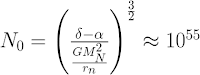Lots of neutrons.

So by modifying an equation used to predict the mass of nuclei with 1 to 300 neutrons, we have derived a result of $10^{55}$ neutrons to be gravitationally stable. This gives a mass of about $10^{28}$ kg or about 1% the mass of the sun.

Now, stellar-sized gravitationally bound agglomerates of neutrons already sort of exist; they are called neutron stars. They are not 100% neutrons; they have a crust that is still full of protons. The interior structure and composition of a neutron star are also not fully known (it's not even known whether their radius gets bigger or smaller as a function of mass), and the core is basically the "here be dragons" of stellar astrophysics. What is known, however, is that a neutron star must be at least 1.44 times the mass of the sun (the Chandrasekhar limit), because below that it can still be supported by electron degeneracy pressure, and the star is a white dwarf.

The gravitational neutron drop model underpredicted the minimum neutron star size by two orders of magnitude. I still think it's impressive, however, that we extrapolated the model by 53 orders of magnitude beyond its intended use and were only off by another two orders.

Neutron Stars

At this point in the post I'll mention that this calculation was not my idea. I saw it in a talk by John Michael Pearson at McGill in 2014. It was a nuclear talk about stars, which is good because nuclear talks about nuclear physics and astrophysics talks about astrophysics are both too technical for a general physicist, but when someone has to talk to people outside their own speciality, the talks become more accessible. In his talk he introduced this calculation, and then went on to refine it to get a better answer. In particular, he was interested in using this type of reasoning and precise nuclear mass data to derive an upper-bound to the neutron star mass (before it collapses into a black hole), which could be compared to observations of very large neutron stars to verify their description of the nuclear physics. He mentioned in the talk that the recent discovery of a very large neutron star had already ruled out one of his models.

He has a paper on it here, and he got $10^{56}$ neutrons (not sure where our divergence lies, perhaps in a factor of 3/5 that I dropped, or maybe I have to take into account the surface terms), within one order of magnitude of the Chandrasekhar limit, before refining his calculation with nuclear physics that is far beyond what I have encountered.

The way neutron star models are typically derived is by constraining the internal density of the star to the pressure by assuming hydrostatic equilibrium, and further constraining the pressure to the density using nuclear physics***. Because gravitational fields in neutron stars are so intense it becomes necessary to make general relativistic corrections to the hydrostatic equilibrium, so it gets pretty complicated.

So, just to summarize, by adding a gravitational attraction to the liquid drop model, you can make a wild extrapolation and get logarithmically-almost the correct answer, which I think is cool.

*I have read mixed conventions of whether the binding energy is additive and negative, or subtractive and positive. The sentence "adding a negative binding energy reduces the mass and makes the nucleus stable, and a larger magnitude of this energy makes it more stable" describes my convention. If this doesn't quite make sense, imagine pulling the protons and neutrons out of the nucleus and considering how that increases the total energy, and then compare the bound energy to the energy of all the particles at infinity, sort of like gravitational potential.  If this still doesn't make sense...leave a comment.

**Sort of like the van der Waals version of the strong nuclear force. There's a misconception that the strong force holds nucleons together, but it actually holds quarks together inside nucleons, and the residual force holds nucleons together.

***I'm now talking about something I don't really understand so it sounds vague.

1.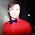Fantastic! Very interesting.

1.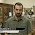2.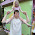Wow. Thanks for this. Just two orders of magnitude is neat, wouldn't have guessed.

1.As a few people on reddit pointed out, the calculation I'm discussing doesn't necessarily point at the neutron star minimum mass, and that neutron stars have the additional constraint of overcoming electron degeneracy before they start expunging themselves of protons. So, it's a perfectly cromulent calculation, it's just calculating something different than the Chandrasekhar mass.

3.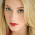This comment has been removed by a blog administrator.